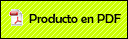# CONTINUOUS AND DISCRETE FLOWS ON OPERATOR ALGEBRAS

###Itzá Ortiz, Benjamín Alfonso2009

Itzá-Ortiz, B., Continuous and discrete flows on operator algebras, Journal of the Australian Mathematical Society 86 (2009), 169--176. Preprinted

Abstract

Let (N, R, ) be a centrally ergodic W* dynamical system. When N is not a factor, we show that, for each t 6= 0, the crossed product induced by the time t automorphism t is not a factor if and only if there exist a rational number r and an eigenvalue s of the restriction of to the center of N, such that rst = 2. In the C* setting, minimality seems to be the notion corresponding to central ergodicity. We show that if (A, R, ) is a minimal unital C* dynamical system and A is either prime or commutative but not simple, then, for each t 6= 0, the crossed product induced by the time t automorphism t is not simple if and only if there exist a rational number r and an eigenvalue s of the restriction of to the center of A, such that rst = 2.Matematicas en la distribucion espacial de poblaciones

Multichannel Detrended Fluctuation Analysis Reveals Synchronized Patterns of Spontaneous Spinal Acti...

D-Branes in Orientifolds and Orbifolds and Kasparov KK-Theory

Una Conjetura de Polya y Szego para el Tono Fundamental de Membranas Poligonales

THE C*-ALGEBRAS ASSOCIATED TO TIME-t AUTOMORPHISMS OF MAPPING TORI

Slow decay of end effects in layered structures with an imperfect interface

REALIZATION OF A SIMPLE HIGHER DIMENSIONAL NONCOMMUTATIVE TORUS AS A TRANSFORMATION GROUP C*-ALGEBRA

Propagation of Elastic Waves along Interfaces in Layered Beams

Eigenfunction expansions and spectral projections for isotropic elasticity outside an obstacle

PROPAGATION OF ELASTIC WAVES ALONG INTERFACES IN LAYERED BEAMS